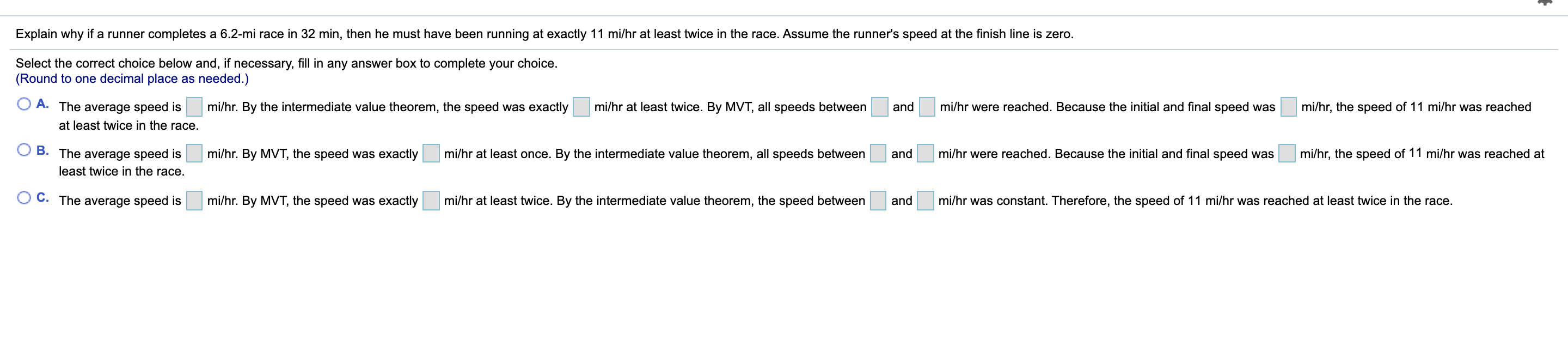# Question Explain why if a runner completes a 6.2-mi race in 32 min, then he must have been running at exactly 11 mi/hr at least twice in the race. Assume the runner's speed at the finish line is zero. Select the correct choice below and, if necessary, fill in any answer box to complete your choice. (Round to one decimal place as needed.) A. mi/hr. By the intermediate value theorem, the speed was exactly mi/hr at least twice. By MVT, all speeds between and mi/hr were reached. Because the initial and final speed was mi/hr, the speed of 11 mi/hr was reached The average speed is at least twice in the race. B. The average speed is least twice in the race. mi/hr. By MVT, the speed was exactly mi/hr at least once. By the intermediate value theorem, all speeds between and mi/hr were reached. Because the initial and final speed was mi/hr, the speed of 11 mi/hr was reached at OC. The average speed is mi/hr. By MVT, the speed was exactly mi/hr at least twice. By the intermediate value theorem, the speed between and mi/hr was constant. Therefore, the speed of 11 mi/hr was reached at least twice in the race.